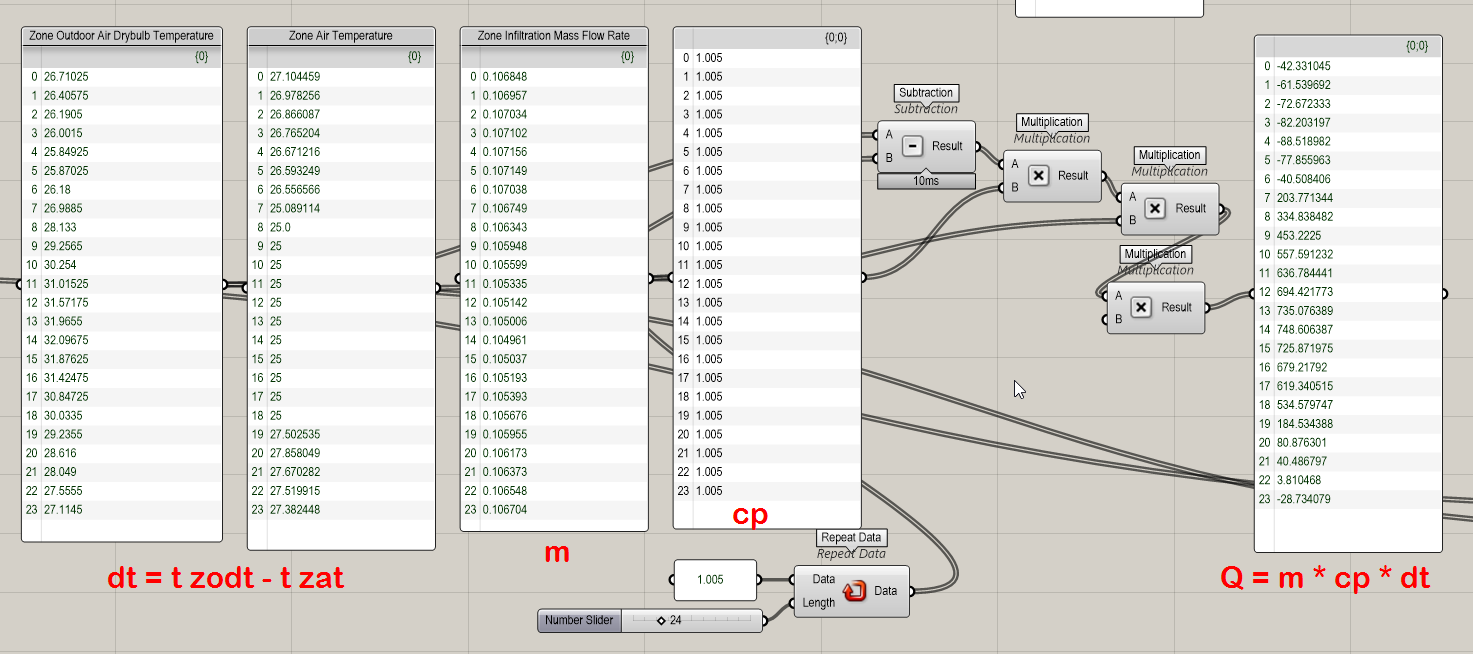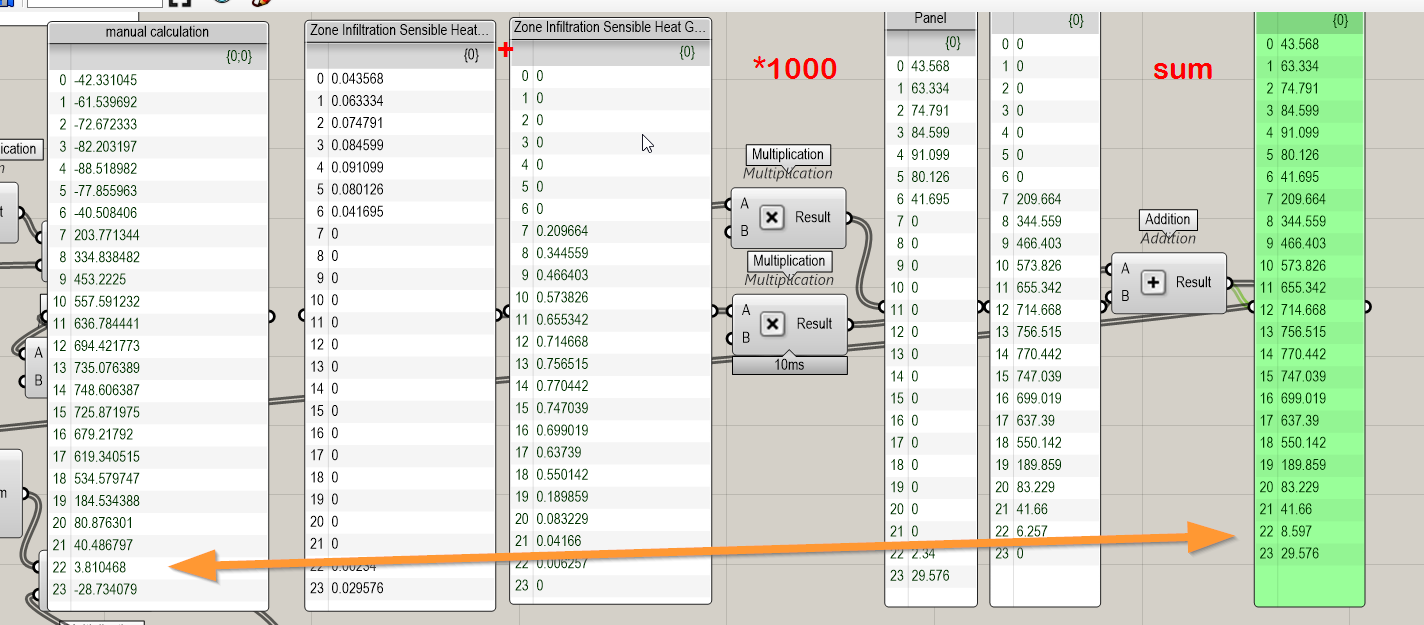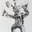Question-and-Answer Resource for the Building Energy Modeling Community
Get started with the Help page

how to find Zone Infiltration Rate? or equivalent in [W]

How to calculate infiltration rate [W] I am trying to get all variables that 'make' design heat loss and design cooling load. I manage to find infiltration mass flow rate from the available reports list:

Zone Infiltration Sensible Heat Loss Energy
Zone Infiltration Sensible Heat Gain Energy
Zone Infiltration Latent Heat Loss Energy
Zone Infiltration Latent Heat Gain Energy
Zone Infiltration Total Heat Loss Energy
Zone Infiltration Total Heat Gain Energy
Zone Infiltration Current Density Volume Flow Rate
Zone Infiltration Standard Density Volume Flow Rate
Zone Infiltration Current Density Volume
Zone Infiltration Standard Density Volume
Zone Infiltration Mass
Zone Infiltration Mass Flow Rate
Zone Infiltration Air Change RateZone Infiltration Sensible Heat Loss Energy and Zone Infiltration Sensible Heat Gain Energy
but this gives me different results...I guess cp is recalculated for each hour? rather than my assumed cp=1.005 kJ/kgK?edit retag close merge delete

Sort by » oldest newest most votedI'm not sure I follow the question. To answer the question in the title, the infiltration gain or loss in W is simply the "Zone Infiltration Sensible Heat Loss Energy [J]" divide by the reporting frequency in seconds.

Are you trying to reproduce the calculations for Zone Infiltration Sensible/Latent Heat Loss/Gain?

If you want to trace a particular output variable, search the source code for the name of the output, and find the corresponding SetupOutputVariable call. (Usually there will be only one hit, but sometimes the output variable names show up in other report setups.)

SetupOutputVariable(state,
"Zone Infiltration Sensible Heat Loss Energy",
OutputProcessor::Unit::J,
state.dataHeatBal->ZnAirRpt(state.dataHeatBal->Infiltration(Loop).ZonePtr).InfilHeatLoss,
OutputProcessor::SOVTimeStepType::System,
OutputProcessor::SOVStoreType::Summed,
state.dataHeatBal->Zone(state.dataHeatBal->Infiltration(Loop).ZonePtr).Name);

The 3rd argument is the variable associated with this output, ZnAirRpt.InfilHeatLoss. Searching on InfilHeatLoss leads to:

Infiltration(j).InfilHeatLoss = Infiltration(j).MCpI_temp * (state.dataHeatBalFanSys->MAT(NZ) - Zone(NZ).OutDryBulbTemp) * TimeStepSys *

MCpI_temp = IVF * AirDensity * CpAir *
(state.dataHeatBal->Infiltration(j).ConstantTermCoef +
std::abs(TempExt - state.dataZoneEquip->ZMAT(NZ)) * state.dataHeatBal->Infiltration(j).TemperatureTermCoef +
WindSpeedExt * (state.dataHeatBal->Infiltration(j).VelocityTermCoef +
WindSpeedExt * state.dataHeatBal->Infiltration(j).VelocitySQTermCoef));

And a few lines above that:

AirDensity = PsyRhoAirFnPbTdbW(state, state.dataEnvrn->OutBaroPress, TempExt, HumRatExt, RoutineNameInfiltration);
CpAir = PsyCpAirFnW(HumRatExt);

The air properties are based on outdoor conditions.

more